# 4.5 Discussion and Exercises

Skiplists were introduced by Pugh  who also presented a number of applications and extensions of skiplists . Since then they have been studied extensively. Several researchers have done very precise analyses of the expected length and variance of the length of the search path for theth element in a skiplist [45,44,58]. Deterministic versions , biased versions [8,26], and self-adjusting versions  of skiplists have all been developed. Skiplist implementations have been written for various languages and frameworks and have been used in open-source database systems [71,63]. A variant of skiplists is used in the HP-UX operating system kernel's process management structures . Skiplists are even part of the Java 1.6 API .

Exercise 4..1   Illustrate the search paths for 2.5 and 5.5 on the skiplist in Figure 4.1.

Exercise 4..2   Illustrate the addition of the values 0.5 (with a height of 1) and then 3.5 (with a height of 2) to the skiplist in Figure 4.1.

Exercise 4..3   Illustrate the removal of the values 1 and then 3 from the skiplist in Figure 4.1.

Exercise 4..4   Illustrate the execution of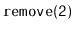on the SkiplistList in Figure 4.5.

Exercise 4..5   Illustrate the execution of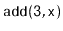on the SkiplistList in Figure 4.5. Assume thatselects a height of 4 for the newly created node.

Exercise 4..6   Show that, during an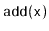or a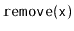operation, the expected number of pointers in a SkiplistSet that get changed is constant.

Exercise 4..7   Suppose that, instead of promoting an element from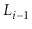intobased on a coin toss, we promote it with some probability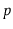,.
1. Show that, with this modification, the expected length of a search path is at most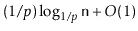.
2. What is the value ofthat minimizes the preceding expression?
3. What is the expected height of the skiplist?
4. What is the expected number of nodes in the skiplist?

Exercise 4..8   The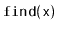method in a SkiplistSet sometimes performs redundant comparisons; these occur whenis compared to the same value more than once. They can occur when, for some node,,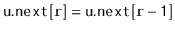. Show how these redundant comparisons happen and modifyso that they are avoided. Analyze the expected number of comparisons done by your modifiedmethod.

Exercise 4..9   Design and implement a version of a skiplist that implements the SSet interface, but also allows fast access to elements by rank. That is, it also supports the function, which returns the element whose rank is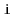inexpected time. (The rank of an element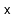in an SSet is the number of elements in the SSet that are less than.)

Exercise 4..10   A finger in a skiplist is an array that stores the sequence of nodes on a search path at which the search path goes down. (The variablein thecode on pageis a finger; the shaded nodes in Figure 4.3 show the contents of the finger.) One can think of a finger as pointing out the path to a node in the lowest list,.

A finger search implements theoperation using a finger, by walking up the list using the finger until reaching a nodesuch thatand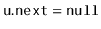orand then performing a normal search forstarting from. It is possible to prove that the expected number of steps required for a finger search is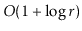, whereis the number values in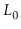betweenand the value pointed to by the finger.

Implement a subclass of Skiplist called SkiplistWithFinger that implementsoperations using an internal finger. This subclass stores a finger, which is then used so that everyoperation is implemented as a finger search. During eachoperation the finger is updated so that eachoperation uses, as a starting point, a finger that points to the result of the previousoperation.

Exercise 4..11   Write a method,, that truncates a SkiplistList at position. After the execution of this method, the size of the list isand it contains only the elements at indices. The return value is another SkiplistList that contains the elements at indices. This method should run intime.

Exercise 4..12   Write a SkiplistList method,, that takes as an argument a SkiplistList,, empties it and appends its contents, in order, to the receiver. For example, ifcontains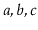and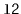contains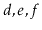, then after calling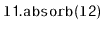,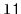will contain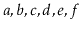andwill be empty. This method should run intime.

Exercise 4..13   Using the ideas from the space-efficient list, SEList, design and implement a space-efficient SSet, SESSet. To do this, store the data, in order, in an SEList, and store the blocks of this SEList in an SSet. If the original SSet implementation uses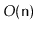space to store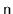elements, then the SESSet will use enough space forelements pluswasted space.

Exercise 4..14   Using an SSet as your underlying structure, design and implement an application that reads a (large) text file and allows you to search, interactively, for any substring contained in the text. As the user types their query, a matching part of the text (if any) should appear as a result.

Hint 1: Every substring is a prefix of some suffix, so it suffices to store all suffixes of the text file.

Hint 2: Any suffix can be represented compactly as a single integer indicating where the suffix begins in the text.

Test your application on some large texts, such as some of the books available at Project Gutenberg . If done correctly, your applications will be very responsive; there should be no noticeable lag between typing keystrokes and seeing the results.

Exercise 4..15   (This exercise should be done after reading about binary search trees, in Section 6.2.) Compare skiplists with binary search trees in the following ways:
1. Explain how removing some edges of a skiplist leads to a structure that looks like a binary tree and is similar to a binary search tree.
2. Skiplists and binary search trees each use about the same number of pointers (2 per node). Skiplists make better use of those pointers, though. Explain why.

opendatastructures.org